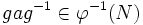# Normality satisfies inverse image condition

This article gives the statement, and possibly proof, of a subgroup property satisfying a subgroup metaproperty
View all subgroup metaproperty satisfactions | View all subgroup metaproperty dissatisfactions |Get help on looking up metaproperty (dis)satisfactions for subgroup properties
|

## Statement

### Property-theoretic statement

The subgroup property of being normal satisfies the subgroup metaproperty called the inverse image condition: the inverse image of a normal subgroup, under a homomorphism, is normal.

### Statement with symbols

Let$\varphi:G \to H$ be a homomorphism of groups, and$N$ be a normal subgroup of$H$. Then,$\varphi^{-1}(N)$ is a normal subgroup of$G$.

## Proof

Given:$\varphi:G \to H$, a homomorphism of groups, and$N$ is a normal subgroup of$H$

To prove:$\varphi^{-1}(N)$ is normal in$G$

Proof: Pick$a \in \varphi^{-1}(N)$ and$g \in G$. We need to show that$gag^{-1} \in \varphi^{-1}(N)$.

By the fact that$\varphi$ is a homomorphism:$\varphi(gag^{-1}) = \varphi(g)\varphi(a)\varphi(g)^{-1}$

Since$a \in \varphi^{-1}(N)$,$\varphi(a) \in N$, and since$N$ is normal in$H$, the right side of the above equation is in$N$. Hence,$\varphi(gag^{-1}) \in N$, so$gag^{-1} \in \varphi^{-1}(N)$, as required.